Courses

# Test: Previous Year Questions: Coordination Compounds

## 22 Questions MCQ Test Chemistry Class 12 | Test: Previous Year Questions: Coordination Compounds

Description
This mock test of Test: Previous Year Questions: Coordination Compounds for JEE helps you for every JEE entrance exam. This contains 22 Multiple Choice Questions for JEE Test: Previous Year Questions: Coordination Compounds (mcq) to study with solutions a complete question bank. The solved questions answers in this Test: Previous Year Questions: Coordination Compounds quiz give you a good mix of easy questions and tough questions. JEE students definitely take this Test: Previous Year Questions: Coordination Compounds exercise for a better result in the exam. You can find other Test: Previous Year Questions: Coordination Compounds extra questions, long questions & short questions for JEE on EduRev as well by searching above.
QUESTION: 1

### In [Cr(C2O4)3]3_ , the isomerism shown is [AIEEE-2002]

Solution:

The correct answer is B.
[Cr(Ox)3​]3−  Ox = C2O42−

Optical Isomer ⟶ Non-superimposable mirror images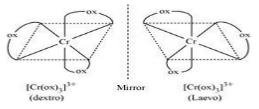QUESTION: 2

### In the complexes [Fe(H2O)6]3+, [Fe(CN)6]3+, [Fe(C2O4)3]3_ and [FeCl6]3_, more stability is shown by -

Solution:

The correct answer is Option C.

The central metal ion is Fe3+ and C2O42-
is a negative bi-dentate ligand which forms a more stable complex than neutral or monodentate ligand.
Metal chelates are more stable than non-chelates. oxalate is a bidentate ligand and forms metal chelates.
Hence,[Fe(C2​O4​)3​]3− is more stable.

QUESTION: 3

### One mole of the complex compound Co(NH3)5Cl3, gives 3 moles of ions on dissolution in water. One mole of the same complex reacts with two moles of AgNO3solution to yield two moles of AgCl(s). The structure of the complex is –    [AIEEE-2003]

Solution: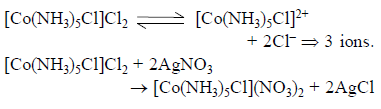QUESTION: 4

In the coordination compound K4[Ni(CN)4], the oxidation state of nickel is –

[AIEEE-2003]

Solution:

The correct answer is option A
Consider the compound K4​[Ni(CN)4​]
Let oxidation state of Nickel be x
(1×4)+x+(4×−1)=0
⇒ 4+x−4=0
⇒ x=0
Hence, in the given compound, the oxidation state of Nickel is zero.

QUESTION: 5

The number of 3d-electrons remained in Fe2+ (At. no. of Fe = 26) is –

[AIEEE-2003]

Solution:

The correct answer is option C
Fe (26)
Electronic configuration: 1s2 2s2 2p6 3s2 3p6 3d5 3p6 4s2
Fe2+  Electronic configuration: 1s22s22p6 3s23p63d53p64s0
6  d-electrons are retained in Fe2+.

QUESTION: 6

Ammonia forms the complex ion [Cu(NH3)4]2+ ion with copper ions in alkaline solutions but not in acidic solution. What is the reason for it –

[AIEEE-2003]

Solution:

The correct answer is option B
In acidic solutions, ammonia reacts with proton and forms ammonium ion which further cannot act as a ligand. Also, no free ammonia molecules are available for co-ordination. Hence, it does not form a complex with copper ion.
The reaction is as follows:
NH3​ + H+ → NH4+

QUESTION: 7

Among the properties (a) reducing (b) oxidising (c) complexing, the set of properties shown by CN_ ion towards metal species is –

[AIEEE-2004]

Solution: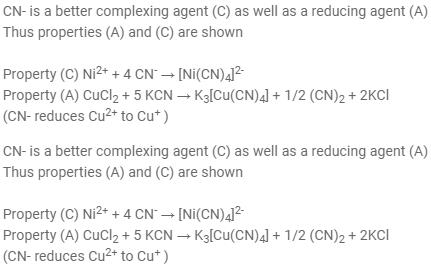Thus correct option is A

QUESTION: 8

The coordination number of a central metal atom in a complex is determined by –

[AIEEE-2004]

Solution:

The correct answer is Option C.
Coordination number is the maximum covalency shown by a metal or metal ion. lt is the maximum number of ligands attached to the metal by sigma bonds or coordinate bonds.
Here, the ligand is monodentate and attached to the metal via sigma bond.

QUESTION: 9

Which one of the following complexes in an outer orbitals complex–

[AIEEE-2004]

Solution:

The correct answer is option D
Generally weaker field ligands form outer orbital complexes.
CN− is a very strong ligand and NH3​ is a weak ligand.
So [Co(NH3​)6​]3+ and  [Ni(NH3​)6​]2+ can form an outer orbital complex.
Electronic configuration of Co+3 is [Ar]3d64s0
Electronic configuration of Ni+2 is [Ar]3d84s0
Nickel complexes can form due to presence of d8 configuration.

QUESTION: 10

Coordination compounds have great importance in biological systems. In this contect which of the following statements is incorrect ?

[AIEEE-2004]

Solution:

Chlorophylls are green pigments in plants and contain magnesium.
They are useful during photosynthesis to store energy in the form of glucose from carbon dioxide and water in presence of sunlight.

QUESTION: 11

The correct order of magnetic moments (spin only values in B.M. among is) –

[AIEEE-2004]

Solution:

The correct answer is option C
Cl is a weak ligand while CN is a strong ligand.
In [MnCl4​]2: d5 configuration and 5 unpaired electrons
In [CoCl4​]2: d7 configuration and 3 unpaired electrons
In [Fe(CN)6​]4: d6 configuration, low spin complex and 0 unpaired electrons
More the number of unpaired electrons, more the value of magnetic moment. Hence, the order is: [MnCl4​]2−>[CoCl4​]2−>[Fe(CN)6​]4−

QUESTION: 12

The value of the 'spin only' magnetic moment for one of the following configurations is 2.84 BM . The correct one is –

[AIEEE-2005]

Solution:

Spin only magnetic moment = √(n(n+2)) B.M.

Where n = number of unpaired electron

Given, √(n(n+2)) = 2.84

n(n + 2) = 8.0656

n = 2

In an octahedral complex, for a d4 configuration in a strong field ligand, number of unpaired electrons = 2

Hence A is correct.

QUESTION: 13

The IUPAC name for the complex [Co(NO2)(NH3)5] Cl2 is –

[AIEEE-2006]

Solution:

The correct option is B.
The IUPAC name of the given coordination complex according to the nomenclature rules is Pentamminenitrito-N-cobalt(II)chloride.

QUESTION: 14

Nickel (Z = 28) combines with a ninegative monodentate ligand X_ to form a paramagnetic complex [NiX4]2_. The number of unpaired electron in the nickel and geometry of this complex ion are respectively –

[AIEEE-2006]

Solution:

The correct answer is option D
Two,tetrahedral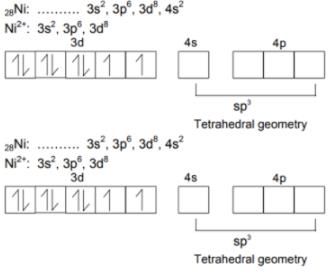QUESTION: 15

In Fe(CO)5, the Fe–C bond possesses –

[AIEEE-2006]

Solution:

The correct answer is option D
(Both s and p character)
It's called “synergic bonding”. The ligand (CO) donates it's lone pair of electrons to the vacant orbitals of the iron atom and forms the sigma-bond. Since the iron atom also possesses some electrons in its d-orbitals, it back donates those electrons to the molecular orbitals of the ligand forming a π-bond. In this way, the metal-carbon bond length is reduced and the complex gets more stability. One important thing to keep in mind is that the metal atom donates its electron pairs to the antibonding MO of CO, so the C-O bond is weakened by this synergic bonding, leading to a larger C-O bond length in the complex (as opposed to a free CO molecule).
Metal-C bond length reduces, C-O bond length increases, complex gets stability.

QUESTION: 16

How many EDTA (ethylenediaminetetraacetate ion) molecules are required to make an octahedral complex with a Ca2+ ion ?

[AIEEE-2006]

Solution:

The correct answer is option A

An octahedral complex has 6 bonds around the central atom . One EDTA moledule complexes with one molecule of calcium cation to form an octahedral complex.

QUESTION: 17

The ''spin-only'' magnetic moment [in units of Bohr magneton] of Ni2+ in aqueous solution would be (At. No. Ni = 28) –

[AIEEE-2006]

Solution:

The correct answer is option C
In aqueous solution, as aqua is poor ligand so high spin complex forms.
Electronic configuration of Ni=[Ar] 4s23d8
Electronic configuration of Ni2+=[Ar] 4s0 3d8
Ni+2,d8 configuration, have 2 unpaired electrons so the magnetic moment is: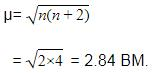QUESTION: 18

Which one of the following has a square planar geometry - (Co = 27, Ni = 28, Fe = 28, Fe = 26, Pt = 78) –

[AIEEE-2007]

Solution:

The correct answer is Option D.
Cl- is weak field ligand and so chloride complexes are mostly high spin, but CFSE for transition metals is in the order 3d<4d<5d , so the metal belonging to higher series will prefer low spin complex. Among the given complexes, Pt forms a low spin complex while others form a high spin complex. Square planar geometry of [PtCl4]2− is also supported by its diamagnetic nature.
Note: All the complexes of Pd (II) and Pt (II) are square planar.

QUESTION: 19

The coordination number and the oxidation state of the element 'E' in the complex [E(en)2(C2O4)] NO2 (where (en) is ethylene diamine) are, respectively –

[AIEEE-2008]

Solution:

The correct answer is option D
The coordination number is the number of coordinate covalent bonds formed with a central metal atom. ligand en is a bidentate ligand that has two donor atoms in one molecule. So, when two en ligands attach to the central atom they will make four covalent coordinate bonds. Also, Oxalate (C2​O4​) is bidentate ligand making two coordinate covalent bonds at a time. Hence overall the coordination number of the element E is 4+2=6.
The oxidation number can be calculated by adding the charges of all the ligands and counter ions (ions present outside the central atom).
Let the oxidation state of element E be x.
Ligand en is neutral. Oxalate carries−2charge NO2​ (counter ion) carries −1charge.

So,  x + (2 × 0) + (−2) + (−1) = 0
x − 2 − 1 = 0
x = +3

QUESTION: 20

In which of the following octahedral complexes of Co (at. no. 27), will the magnitude of Dbe the highest ?

Solution:

The correct answer is Option A.
∆о is the magnitude of splitting of d-orbitals in the octahedral field. Strong field ligands cause large splitting of d-orbitals into t2g and eg orbitals where t2g has lower energy than d-orbitals and eg has higher energy than d-orbitals.
Spectrochemical series is the series in which ligands are arranged in the order of their increasing splitting ability. The CN- ligands are at the right most end indicating the high splitting power.

QUESTION: 21

Which of the following has an optical isomer ?

[AIEEE-2009]

Solution:

The correct answer is Option C.
[CO(en)2(NH3)2]3+ doesn’t have a plane of symmetry and shows optical isomerism.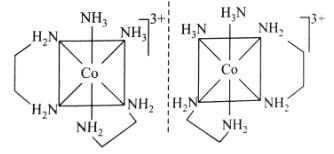QUESTION: 22

Which of the following pairs represents linkage isomers ?

[AIEEE-2009]

Solution:

The correct answer is Option A
Linkage isomerism is shown by ambidentate ligands like NCS and SCN.
It can  be linked through N( or ) S
∴[Pd(PPh3)(NCS2)] and [Pd(PPh3)2(SCN)2